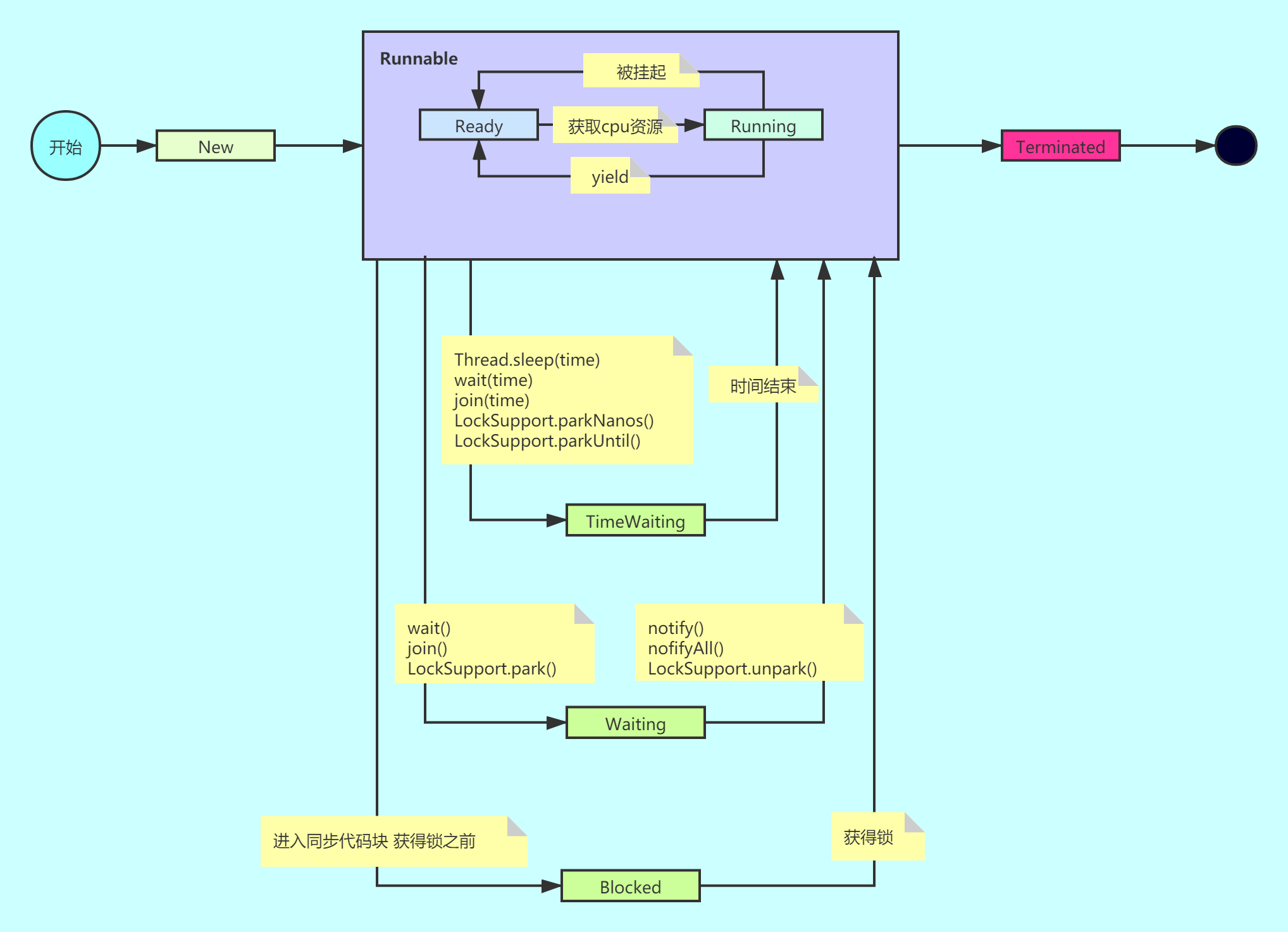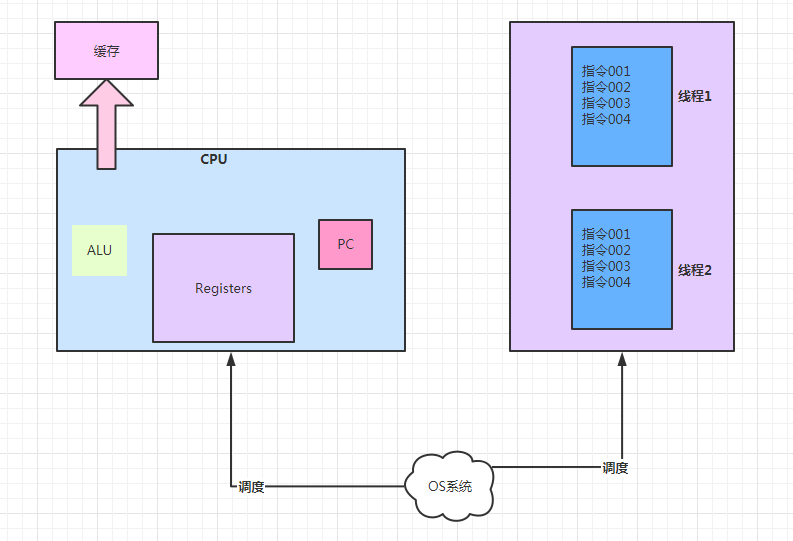# 话说 线程切换 & 线程数设置#### 一、 线程切换1. 操作系统让 cpu 执行线程 1

2. 线程 1 执行到指令 003 的时候 操作系统让 cpu 执行线程 2

3. cpu 会将线程 1 的执行半成品放到缓存中

4. cpu 接着执行线程 2

5. cpu 执行线程 2 的 004 指令的时候 操作系统又让 cpu 执行线程 1

6. cpu 从缓存拿出线程 1 的残次品接着执行

7. cpu 就是一个无脑的计算中心 它不管调度 只管计算（像极了我们程序员）

8. 而操作系统 是负责调度的 （像极了我们的领导）

9. 在这里应该能发现一个点那就是：线程的切换是需要耗费操作系统资源的 （领导使唤你干活儿 肯定要浪费领导时间和精力）

#### 二、 单核 cpu 设置多线程 是否有意义

CPU 密集型：

​ CPU 密集型也叫计算密集型，简单说就是大部分时候 CPU 使用在 100% ，而读写磁盘/内存时间很短

​ 比如大量数据计算的，进行数据分析的 ，也可能是 CPU 性能极差的而磁盘/内存性能很好的情况

IO 密集型：

​ IO 密集型跟 CPU 密集型相反，大部分时间 CPU 使用率很小，而读写磁盘/内存占用很大时间

​ 比如读数据库比较多的，网络请求比较多的，也可能是磁盘/内存质量差的的情况

#### 三、 线程数越大越好吗

public class NumTest {    // 一有1亿条数据的数组    public static  double[] arr = new double;    public static void main(String[] args) throws InterruptedException {        // 初始化        Random r = new Random();        for (int i = 0; i < arr.length; i++) {            arr[i] =r.nextInt(10);        }        // 单线程计算        getSum01();        // 2线程计算        getSum02();        // 多个线程计算        getSum03();    }    // 单线程计算sum    private static void getSum01() {        long start = System.currentTimeMillis();        double sum = 0;        // 遍历求和        for (int i = 0; i <arr.length ; i++) {            sum = sum+arr[i];        }        long end = System.currentTimeMillis();        // 输出日志        System.out.println("一个线程计算 耗时:"+(end-start) + "毫秒，"  +"  计算结果:"+sum);    }    /**     * 2个线程计算sum     */    static double sum01,sum02;    private static void getSum02() throws InterruptedException {        // 第一个线程 计算0到一半        Thread thread01 = new Thread(() -> {            for (int i = 0; i < arr.length / 2; i++) {                sum01 = sum01 + arr[i];            }        });        // 第二个线程 计算一半到length        Thread thread02 = new Thread(() -> {            for (int i = arr.length / 2; i < arr.length; i++) {                sum02 = sum02 + arr[i];            }        });        long start = System.currentTimeMillis();        thread01.start();        thread02.start();        // join 到主线程  阻塞        thread01.join();        thread02.join();        // 执行完之后 求一下两个线程分别求和的和        double  sum =sum01+sum02;        long end = System.currentTimeMillis();        // 打印日志        System.out.println("2个线程计算 耗时:"+(end-start) + "毫秒，"  +"  计算结果:"+sum);    }    /**     * 多个线程计算     * @throws InterruptedException     */    private static void getSum03() throws InterruptedException {        // 10个线程 可自行调节        int ThreadNum = 1000;        // 创建ThreadNum个长度的long数组 存储各个线程的求和结果        double[] sums = new double[ThreadNum];        // 存储多个线程        Thread[]  threads = new Thread[ThreadNum];        // 每个线程计算的数字个数        final int segCount = arr.length/ThreadNum;        CountDownLatch cdl = new CountDownLatch(ThreadNum);        // 创建多个线程        for (int i = 0; i < ThreadNum; i++) {            int m = i;            Thread thread = new Thread(() -> {                // 每个线程执计算逻辑                for (int j = segCount*m; j <segCount*(m+1) && j < arr.length; j++) {                    sums[m]+=arr[j];                }                cdl.countDown();            });            threads[i] = thread;        }        long start = System.currentTimeMillis();        // 全部启动        for (int i = 0; i < threads.length; i++) {            threads[i].start();        }        cdl.await();        // 最后求和        double sum = 0;        for (int i = 0; i < sums.length; i++) {            sum+=sums[i];        }        long end = System.currentTimeMillis();        // 打印日志        System.out.println("多个线程计算 耗时:"+(end-start) + "毫秒，"  +"  计算结果:"+sum);    }}输出结果：一个线程计算 耗时:177毫秒，  计算结果:4.50063291E82个线程计算 耗时:99毫秒，  计算结果:4.50063291E8多个线程计算 耗时:162毫秒，  计算结果:4.50063291E8

#### 四、 线程设置多少合适呢

1. 实践中多少都是通过压测来确定的 但是压测初始值是可以自己推算一个的

2. 怎么推算 比如我刚才那个例子： 我电脑 4 核 我想让每个核一个线程这样切换就少了 我把 ThreadNum 设置为 4

3. 输出结果：

一个线程计算 耗时:177毫秒，  计算结果:4.49997398E82个线程计算 耗时:99毫秒，  计算结果:4.49997398E8多个线程计算 耗时:79毫秒，  计算结果:4.49997398E8

1. 那我们有多少核就设置多少个线程？不是的！首先，cpu 不是只为你一个人服务的 除了你还有很多别的进程需要 cpu 一般说的充分利用 CPU 不是说都用到 100% 我们还要留一部分 cpu 进行利用，跟你上班一样，地铁 30 分钟+走路 10 分钟到公司，你如果 9 点上班 你会 8 点 20 出门吗 肯定会早一点出门 留点儿余地

2. 线程数量 设定公式 Nthread = Ncpu * Ucpu * (1+W/C )Ncpu : 处理器的核数 java 可以这样获取：

    System.out.println(Runtime.getRuntime().availableProcessors());

Ucpu: 期望 CPU 的利用率 在 0 到 1 之间

W/C: 是等待时间与计算时间的比率 wait/compute

​ 如果 c(计算时间)占比很大 也就是 w/c ≈ 0 那合适的线程数就等于 NcpuxUcpux1

1. 4 中说的 w 和 c 我怎么知道的 ？这些值呢，一般来说 通过工具(profiler)进行测算

2. java 最常用的是 JProfiler (收费的) 本地开发测试

3. 如果已经部署到服务器上了 可以用阿里开源的 Arthas 做统计

4. 后边会简单写一下:JProfiler Arthas 的使用## 评论